# Taylor integration of the Kepler problem¶

Here, we try to reproduce exactly the Kepler problem integration example made by Luis Benet in JuliaDiff/TaylorSeries.jl.

The Kepler problem is the basis of planetary motion; it describes the motion of a secondary body (e.g., a planet, asteroid, comet, etc.), around a primary body (e.g., the Sun). In cartesian coordinates over the orbital plane, the Hamiltonian for the Kepler problem reads:

\begin{align} H_{\mathrm{Kepler}} &= \frac{1}{2\mu}(p_x^2+p_y^2)-\frac{\mu}{\sqrt{x^2+y^2}} \end{align}

where $\mu=G(m_1+m_2)$, $G$ is the gravitational constant, $m_1$ is the mass of the primary body and $m_2$ is the mass of the secondary body. If we write $\vec r_1 = (x_1,y_1)$ and $\vec r_2 = (x_2,y_2)$, respectively, for the position of the primary and secondary body, then the vector $\vec r = \vec r_2-\vec r_1$, with coordinates $\vec r = (x, y)=(x_2-x_1,y_2-y_1)$, represents the position of the secondary body relative to the primary body; i.e., $\vec r$ represents the so-called relative coordinates. In terms of the vector $\vec{r}$, the position $\vec{r}=0$ corresponds to the position of the primary body.

Using Hamilton equations, we can obtain the equations of motion for the Kepler problem:

\begin{align} \dot x &= u \\ \dot y &= v \\ \dot u &= -\frac{\mu x}{(x^2+y^2)^{3/2}}\\ \dot v &= -\frac{\mu y}{(x^2+y^2)^{3/2}} \end{align}

Note that the canonical momenta are $p_x$ and $p_y$, while $u$ and $v$ are, respectively, the $x$ and $y$ components of the velocity; i.e., $p_x = \mu u$ and $p_y = \mu v$.

First of all, we shall include all relevant packages:

In :
using TaylorIntegration, Plots, LaTeXStrings
pyplot()

Fontconfig warning: ignoring UTF-8: not a valid region tag

Out:
Plots.PyPlotBackend()

Some parameters necessary for the integration:

• $\mu$: the gravitational parameter
• q0: the initial condition (we will select an initial condition which corresponds to elliptical motion)
• order: the order of the Taylor expansion
• t_max: the final time of the integration
• abs_tol: the absolute tolerance
• n_iter: the number of time-steps
In :
const μ = 1.0
const q0 = [0.19999999999999996, 0.0, 0.0, 3.0] # a initial condition for elliptical motion
const order = 28
const t0 = 0.0
const t_max = 10000*(2π) # we are just taking a wild guess about the period ;)
const abs_tol = 1.0E-20
const steps = 500000

Out:
500000

As usual, we write down the equations of motion into a function, which here we will name kepler!. q represents the system state; i.e., the set of values of the dynamical variables at a given instant; dq represents the time derivatives of the components of q.

In :
#an auxiliary array which helps with optimization:
const r_p3d2 = Array{TaylorSeries.Taylor1{Float64}}(1)

#the equations of motion for the Kepler problem:
function kepler!(t, q, dq)
r_p3d2 = (q^2+q^2)^(3/2)

dq = q
dq = q
dq = -μ*q/r_p3d2
dq = -μ*q/r_p3d2

nothing
end

Out:
kepler! (generic function with 1 method)

The Taylor integration:

In :
t, q = taylorinteg(kepler!, q0, t0, 0.01, order, abs_tol, maxsteps=2); #warm-up lap
@time t, q = taylorinteg(kepler!, q0, t0, t_max, order, abs_tol, maxsteps=steps);

 43.060857 seconds (464.94 M allocations: 39.523 GB, 12.87% gc time)


The final state:

In :
t[end]/(2pi), q[end,:]

Out:
(10000.0,[0.2,2.72854e-9,-2.27378e-8,3.0])

Let's extract the values of $x$, $y$, $u$ and $v$ for each time-step:

In :
x, y, u, v = view(q,:,1), view(q,:,2), view(q,:,3), view(q,:,4);


## Orbital motion¶

The initial conditions we selected correspond to a elliptical orbit with a relatively high eccentricity: $e=0.8$ for the initial condition q0=[0.19999999999999996, 0.0, 0.0, 3.0]. How does the motion of the planet/asteroid/comet looks like? Well, let's plot its orbit over the $x-y$ plane (the orbit is shown in blue; the position of the primary body is shown as a yellow dot):

In :
scatter(

[0.0], [0.0],
label = L"\mathrm{Center\; of\; attraction}",
ms=10

)
scatter!(

x[1:75:end], y[1:75:end],
title = L"\mathrm{Orbital\;\;motion}",
xaxis = (L"x", (-2.0, 0.5), -2.0:0.5:2.0),
yaxis = (L"y", (-0.8, 0.8), -2.0:0.5:2.0),
label = L"\mathrm{Keplerian\; ellipse}",
ms=0.5

)

Out: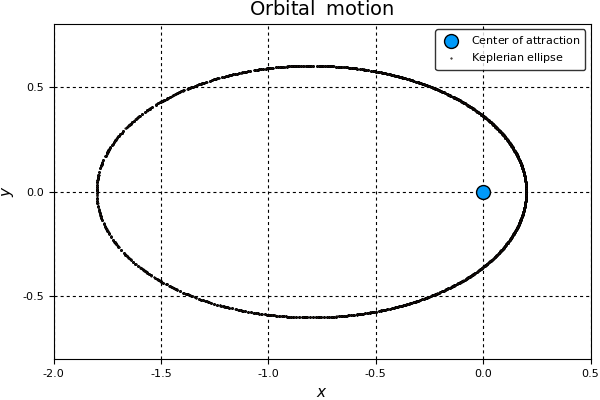## Energy conservation¶

Below, we write the energy function of the Kepler problem; i.e., the Hamiltonian $H_{\mathrm{Kepler}}$ in terms of $x$, $y$, $u$ and $v$:

In :
H(x_, y_, u_, v_) = 0.5*(u_^2+v_^2)-μ/sqrt(x_^2+y_^2)

Out:
H (generic function with 1 method)

Now, using the function H defined above, we calculate the energy during each time-step:

In :
E = H.(x, y, u, v);


$E_0=H_{\mathrm{Kepler}}(x_0,y_0,u_0,v_0)$, the initial value of the energy, is:

In :
E0=E

Out:
-0.5000000000000009

We define $\delta E$ as the relative error in the energy; i.e.:

\begin{align} \delta E(t) &= \frac{E(t)-E_0}{E_0} \end{align}
In :
δE = (E-E0)/(E0)

Out:
436153-element Array{Float64,1}:
-0.0
1.77636e-15
1.77636e-15
8.88178e-16
8.88178e-16
2.66454e-15
3.55271e-15
2.66454e-15
3.55271e-15
2.66454e-15
3.9968e-15
2.66454e-15
3.10862e-15
⋮
-7.12763e-14
-7.19425e-14
-7.14984e-14
-7.10543e-14
-7.19425e-14
-7.10543e-14
-7.10543e-14
-6.92779e-14
-7.01661e-14
-6.92779e-14
-6.92779e-14
-7.10543e-14

The analytical solution preserves the energy; i.e., $\delta E(t)=0$ for the analytical solution. Thus, we expect our solution to be close to zero. So, even if the $\delta E$ is not perfectly zero during the whole integration, it is comparable to Julia's Float64 machine-epsilon.

Below, we plot $\delta E$ in units of Julia's Float64 machine-epsilon as a function of time, $t$. In Julia, the machine-epsilon for the Float64 type has a value

In :
eps(Float64)

Out:
2.220446049250313e-16
In :
plot(

t/(2π), δE/eps(Float64),
title = L"\mathrm{Energy\;\; relative\;\; error\;\; vs\;\; time}",
xaxis = (L"t\mathrm{\,(orbital\;\;periods)}"),
yaxis = (L"\delta E \mathrm{\;\;(machine\;\;epsilons)}"),
label = L"\mathrm{Energy\;\; relative\;\; error\;\; vs\;\; time}"

)

Out: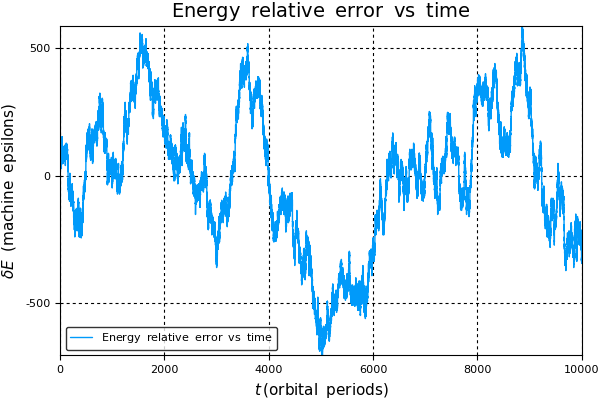Now, how does the energy error distribute around zero?

In :
histogram(

δE/eps(Float64),
title = L"\mathrm{Distribution\;\;of\;\;energy\;\;relative\;\;error}",
xaxis = (L"\delta E"),
yaxis = (L"\mathrm{Number\;\;of\;\;}\delta E\mathrm{\;\;values\;\;within\;\;bin\;\;range}"),
leg = false,
nbins=20

)

INFO: binning = 20

Out: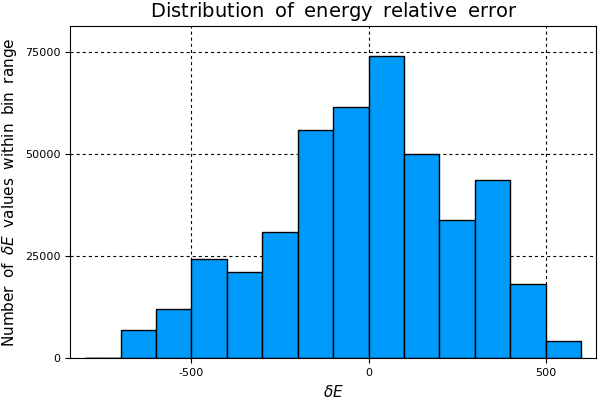The energy error behaves roughly as a random walk, which means that the numerical error in the energy is dominated by rounding errors.

## Angular momentum conservation¶

Analogously to the energy, we now focus on the angular momentum, which is preserved by the analytical solution too. The value of the angular momentum is given by \begin{align} L &= xv-yu \end{align} We write the angular momentum function as:

In :
ang_mom(x_, y_, u_, v_) = x_.*v_-y.*u_

Out:
ang_mom (generic function with 1 method)

So the angular momentum during each time-step is:

In :
L = ang_mom(x, y, u, v);


$L_0=x_0v_0-y_0u_0$, the initial value of the angular momentum, is:

In :
L0 = L

Out:
0.5999999999999999

We define $\delta L$ as the relative error in the angular momentum; i.e.:

\begin{align} \delta L(t) &= \frac{L(t)-L_0}{L_0} \end{align}
In :
δL = (L-L0)/L0;


Just as we did for the energy, we now plot $\delta L$ in units of eps(Float64) vs $t$:

In :
plot(

t/(2π), δL/eps(Float64),
title = L"\mathrm{Angular\;\;momentum\;\; relative\;\; error\;\; vs\;\; time}",
xaxis = (L"t\mathrm{\,(orbital\;\;periods)}"),
yaxis = (L"\delta L \mathrm{\;\;(machine\;\;epsilons)}"),
label = L"\mathrm{Angular\;\;momentum\;\; relative\;\; error\;\; vs\;\; time}",
leg=false,
color=:orange

)

Out: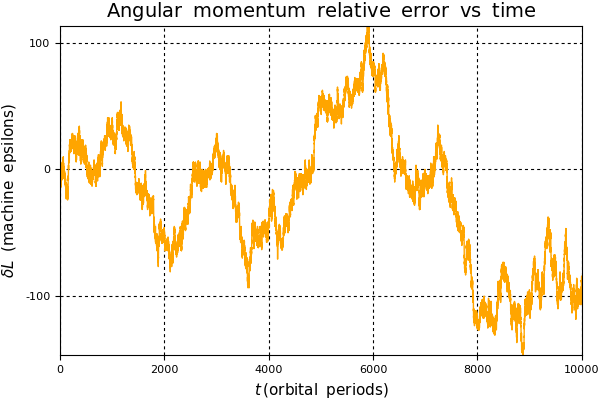Again, we see that the angular momentum relative error is comparable to eps(Float64); the maximum variation is ~$150$ machine epsilons. How does the distribution of this error look like?

In :
histogram(

δL/eps(Float64),
title = L"\mathrm{Distribution\;\;of\;\;angular\;\;momentum\;\;relative\;\;error}",
xaxis = (L"\delta L"),
yaxis = (L"\mathrm{Number\;\;of\;\;}\delta L\mathrm{\;\;values\;\;within\;\;bin\;\;range}"),
leg = false,
nbins=20,
color=:orange

)

INFO: binning = 20

Out: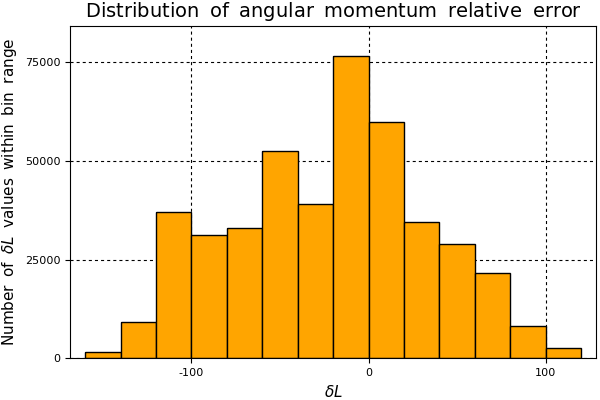The distribution of $\delta L$ shows a peak near zero and roughly symmetrical around this value. This means that the error in the angular momentum is dominated also by rounding errors of floating-point arithmetic.

Lastly, we reproduce exactly the last plot shown in the original version of this example, authored by Luis Benet and included in JuliaDiff/TaylorSeries.jl's Kepler problem integration example jupyter notebook.

A $\delta E$, $\delta L$ plot vs $t$:

In :
plot(

[t/(2π), t/(2π)], [δE/eps(Float64), δL/eps(Float64)],
title = L"\delta E\mathrm{\;\;(blue),\;\;}\delta L\mathrm{\;\;(green)\;\;vs\;\;time}",
xaxis = (L"t\mathrm{\,(orbital\;\;periods)}"),
yaxis = (L"\delta E\mathrm{,\;\;}\delta L\;\;\mathrm{(machine\;\;epsilons)}"),
leg=false,

)

Out: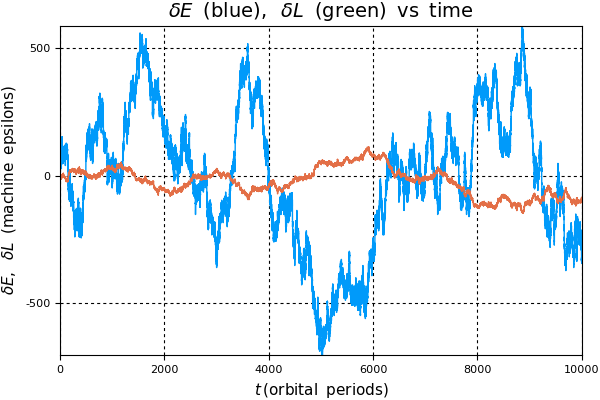In [ ]: